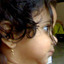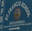Trending ▼   ResFinder# ICSE Class X Prelims 2019 : Mathematics (St. Francis School (SFS), Madhurawada, Visakhapatnam)

1 pages, 2 questions, 0 questions with responses, 0 total responses,00Nag Raj St. Francis School (SFS), Madhurawada, Visakhapatnam
+Fave Message
 Home > nagrajsir >   F Also featured on: School PageFormatting page ...

TEST 18 Question 1 a) If the sum of p terms of and A.P is equal to the sum of q terms, prove that the sum of p+q terms is zero. b) Divide ` 1260 among A,B and C so that the ratio between the shares of A and B is 2:3 and the ratio between the shares of B and C is 4:5 c) Mohan had some shares of face value ` 100 paying 8%dividend. He sold them at ` 130 and invested the proceeds in ` 50 shares of some other company at ` 75 and paying 12% dividend. If by doing so his annual income is increased by ` 360, find the number of shares Mohan had. Question 2 a) The sum of the 11th and 3rd terms of an AP is 34. Find the sum of its 13 terms. b) If x W, find the solution set of 3 5 x 2x 1 3 >1 c) Find the median of the prime numbers between 29 and 60. Question 3 a) Angle A of triangle ABC is right angled. The circle on AC as diameter cuts BC at D. If BD = 9cm, DC = 7cm find the length of AB. b) Govinda opened a recurring deposit account for 2years 4months. The rate of interest is 8% per annum and the bank pays him ` 7369.60 on maturity, find how much per month did govinda deposit? c) two equal cones are touching each other completely at the base circle. Given that the distance between their two vertices is 16cm and the diameter of the base circle is 12cm, find the total surface area of the solid. Question 4 a) In an old story, A thirsty crow found a jar of water cylindrical in shape, filled with some water. As the crow can reach only 5.6cm from the top, the crow wanted to drop pebbles which are spherical in shape, into the jar. If each pebble is 1.4cm in diameter, and the diameter of the jar is 7cm, find how many pebbles should the crow drop to quench its thirst. b) two equal chords AB and CD of a circle with centre O when produced meet at P outside the circle. Prove that (i) PB = PD (ii) PA = PC c) Prove that ( sin + sec )2 + ( cos + cosec )2 = cosec 1+sec Question 5 a) A man wants to buy 124 shares available at ` 66(par value ` 50) (i) How much should he invest (ii) If the dividend is 7.5%, what will be his annual income? (iii) If he wants to increase his income by ` 600 how many extra shares should he buy? b) Two circles touch each other externally at the point P. APC and BPD are straight lines. Show that (i)